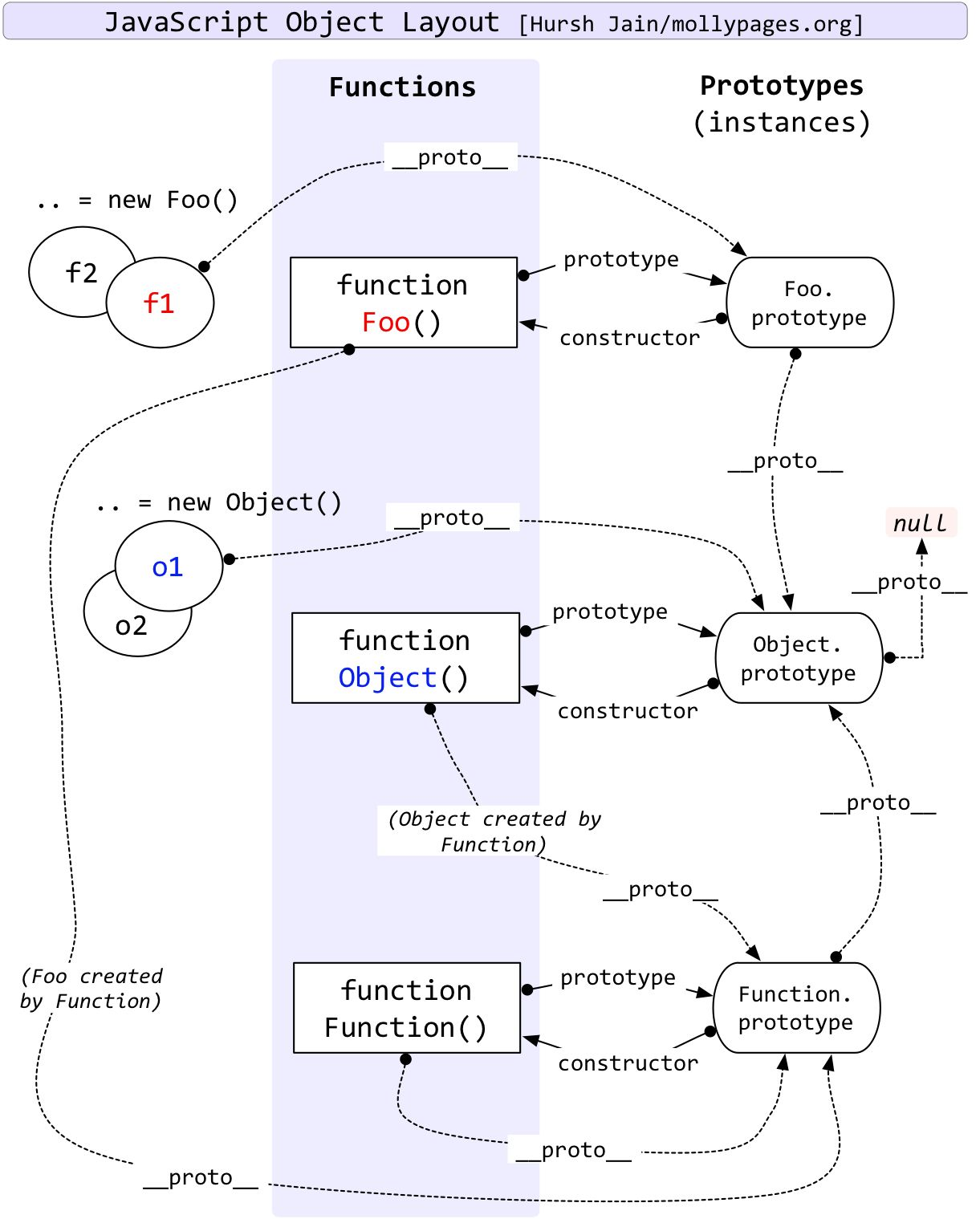# instanceof 和 typeof 的实现原理

## typeof 实现原理

typeof 一般被用于判断一个变量的类型，我们可以利用 typeof 来判断number, string, object, boolean, function, undefined,symbol 这七种类型，这种判断能帮助我们搞定一些问题，比如在判断不是 object 类型的数据的时候，typeof能比较清楚的告诉我们具体是哪一类的类型。但是，很遗憾的一点是，typeof 在判断一个 object的数据的时候只能告诉我们这个数据是 object, 而不能细致的具体到是哪一种 object, 比如

``````let s = new String('abc');
typeof s === 'object'// true
s instanceof String // true
``````

• 000：对象
• 010：浮点数
• 100：字符串
• 110：布尔
• 1：整数

• null：所有机器码均为0
• undefined：用 −2^30 整数来表示

``````null instanceof null // TypeError: Right-hand side of 'instanceof' is not an object

``````

null 直接被判断为不是 object，这也是 JavaScript 的历史遗留bug，可以参考typeof。

``````Object.prototype.toString.call(1) // "[object Number]"
Object.prototype.toString.call('hi') // "[object String]"
Object.prototype.toString.call({a:'hi'}) // "[object Object]"
Object.prototype.toString.call([1,'a']) // "[object Array]"
Object.prototype.toString.call(true) // "[object Boolean]"
Object.prototype.toString.call(() => {}) // "[object Function]"
Object.prototype.toString.call(null) // "[object Null]"
Object.prototype.toString.call(undefined) // "[object Undefined]"
Object.prototype.toString.call(Symbol(1)) // "[object Symbol]"
``````

## instanceof 操作符的实现原理

``````let person = function () {
}
let nicole = new person()
nicole instanceof person // true
``````

``````let person = function () { }
let programmer = function () { }
programmer.prototype = new person()
let nicole = new programmer()
nicole instanceof person // true
nicole instanceof programmer // true

``````

``````function new_instance_of(leftVaule, rightVaule) {
let rightProto = rightVaule.prototype; // 取右表达式的 prototype 值
leftVaule = leftVaule.__proto__; // 取左表达式的__proto__值
while (true) {
if (leftVaule === null) {
return false;
}
if (leftVaule === rightProto) {
return true;
}
leftVaule = leftVaule.__proto__
}
}
``````

``````function Foo() {
}
Object instanceof Object // true
Function instanceof Function // true
Function instanceof Object // true
Foo instanceof Foo // false
Foo instanceof Object // true
Foo instanceof Function // true
``````Object instanceof Object

``````leftValue = Object.__proto__ = Function.prototype;
rightValue = Object.prototype;
// 第一次判断
leftValue != rightValue
leftValue = Function.prototype.__proto__ = Object.prototype
// 第二次判断
leftValue === rightValue
// 返回 true
``````

Function instanceof Function 和 Function instanceof Object 的运行过程与 Object instanceof Object 类似，故不再详说。

Foo instanceof Foo

Foo 函数的 prototype 属性是 Foo.prototype，而 Foo 的 proto 属性是 Function.prototype，由图可知，Foo 的原型链上并没有 Foo.prototype ，因此 Foo instanceof Foo 也就返回 false 。

``````leftValue = Foo, rightValue = Foo
leftValue = Foo.__proto = Function.prototype
rightValue = Foo.prototype
// 第一次判断
leftValue != rightValue
leftValue = Function.prototype.__proto__ = Object.prototype
// 第二次判断
leftValue != rightValue
leftValue = Object.prototype = null
// 第三次判断
leftValue === null
// 返回 false
``````

Foo instanceof Object

``````leftValue = Foo, rightValue = Object
leftValue = Foo.__proto__ = Function.prototype
rightValue = Object.prototype
// 第一次判断
leftValue != rightValue
leftValue = Function.prototype.__proto__ = Object.prototype
// 第二次判断
leftValue === rightValue
// 返回 true
``````

Foo instanceof Function

``````leftValue = Foo, rightValue = Function
leftValue = Foo.__proto__ = Function.prototype
rightValue = Function.prototype
// 第一次判断
leftValue === rightValue
// 返回 true
``````